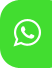## Product Detail## Ignou Solved Assignment For AMT-01/TMA/2019-20

University  IGNOU
Service Type Solved Assignment
Course CTPM
Semester
Short Name or Subject Code Ignou Solved Assignment For AMT-01/TMA/2019-20
Product CTPM of Solved Assignment (IGNOU)
Pattern
Price
Click to view price

1. a) Give one example each, with justification, to support the following statements. (6)

i) For developing mathematical ability, children should pose problems and not merely answer them

ii) Children know some mathematics before they enter formal school.

iii) Children learn by experiencing things.

b) Explain sexagesimal system used for operations on time. Give one example each of the problems involving subtraction and addition of time showing the error children make because of the sexagesimal system. How would you help children correct their error. (6)

c) Draw a number line and mark the following numbers on it. (6) + ,2 −11,− ,5.0,1 + ,5.2 +10 How can you use the number line to show the following

i) −11+10 = −1 ii) 5.2 − 5.0 = 2 d) Give an example each of a theorem and a mathematical conjecture? Justify your examples. (2)

2. a) Explain the process of “generalization” in mathematics, using an example related to understanding volume. Further, explain how this process differs from the process of “Particularization”. (5)

b) Draw pictures to represent the following: (10)

i) 3 2 2 3 ×

ii) 25.0 + 01.0 + 1.0 4

iii) 13 12 8 7 <

iv) 08.0 1.0 >

v) 3 1 of 5

3. a) Explain the meaning of the term “variable”. Write a puzzle for a 10-year child. Write an equation with a variable in it which gives a solution to the question raised by the puzzle. Also give a solution to the puzzle and interpret it. (6)

b) Illustrate by a suitable diagram how to make an angle of 45o by paper folding. (3)

c) i) Identify the possible logic used by a child who gave the following answer. 6 13 2 3 3 2 × = How would you help her clear her misunderstanding.

ii) Suggest an activity to help her understand the algorithm for multiplication of two fractions. (6)

4. a) Describe an activity to help children learn that any line may not be an axis of symmetry of a given circle. Give another activity to assess how far the earlier activity was effective for children to understand the axis of symmetry of a circle. (6)

b) Mathematics learning is not linear, it is spiral in nature. Explain this statement using distinct examples from different areas of Mathematics. (4)

c) Write two examples of situation one from the area of measurement and other from the area of fractions which illustrate the difference between an adult’s and a child’s way of thinking. Describe an activity to minimize the adult-child gap that show up in the primary class room. (5)

5. a) What does “classification” mean? Explain with a suitable example how the ability of “classification” forms a basis for the development of logical and mathematical concept. How does this ability different from the ability of seriation? (6)

b) Explain the difference between language incompetence and cognitive incompetence using examples in the context of learning “angles”. (4)

c) The ability to observe patterns and make a conjecture based on these patterns is an important aspect of mathematical learning. Describe two distinct activities that would help your students to develop this ability. (5)

6. a) How pictorial worksheets can be used to explain the four models of subtraction? (6)

b) Explain the term “concrete operational stage in the context of learning place value”. Do you agree with the statement that most primary school children are in the concrete operational stage. Justify your answer. (4)

7. Which of the following statements are true or false? Give reasons for your answer. (10)

i) In the process of learning a concept, what should be noticed and given recognition is the end product.

ii) Capacity and volume of 3D-objects are different concepts.

iii) The sum of the interior angles of a pentagon is 450o .

iv) All rational numbers are integers but all integers are not rational numbers.

v) 3 (− )4 − (− )7 = − .